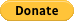# d3-force-bounce

An elastic collision force type for the d3-force simulation engine.

## Usage no npm install needed!

``````<script type="module">
import d3ForceBounce from 'https://cdn.skypack.dev/d3-force-bounce';
</script>``````

# d3.forceBounce

An elastic collision force type for the d3-force simulation engine.

This force is similar to d3.forceCollide, but allows for fully elastic collisions that conserve kinetic energy. This makes d3.forceCollide appropriate for preventing the overlap of nodes, and d3.forceBounce better suited when the intent is to achieve an elastic collision effect, with varying degrees of elasticity (coefficient of restitution).

Here's a visual comparison between the two forces.

It can be used, for example to simulate particle collisions or in a Newton's cradle.

## Quick start

``````import d3ForceBounce from 'd3-force-bounce';
``````

or

``````d3.forceBounce = require('d3-force-bounce');
``````

or even

``````<script src="//unpkg.com/d3-force-bounce/dist/d3-force-bounce.min.js"></script>
``````

then

``````d3.forceSimulation()
.nodes(<myNodes>)
.force('bounce', d3.forceBounce()
);
``````

## API reference

Method Description Default
elasticity([number]) Getter/setter for every collision's coefficient of restitution, aka elasticity. A value of `1` represents a purely elastic collision with no energy loss, while a `0` will fully eliminate the bounce in the collision direction. Values `>1` can be used to introduce acceleration at each collision. Values `<0` are not recommended. 1
radius([num or fn]) Getter/setter for the node object radius accessor function (`fn(node)`) or a constant (`num`) for all nodes. 1
mass([num or fn]) Getter/setter for the node object mass accessor function (`fn(node)`) or a constant (`num`) for all nodes. Mass affects the symmetry of the energy transfer between two colliding nodes. By default it is proportional to the node's area. `Math.pow(radius(node), 2)`
onImpact([fn]) Callback function triggered at every collision, with the signature `onImpact(node1, node2)`

## Giving BackIf this project has helped you and you'd like to contribute back, you can always buy me a ☕!